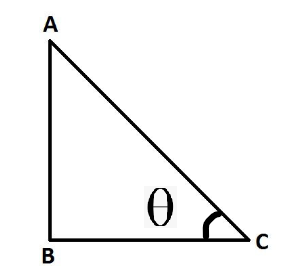Open in App
Not now

# Prove that tan 1°.tan 2°.tan 3° ……… tan 89° = 1

• Last Updated : 03 Sep, 2021

The study of the properties of triangles and their use for the various application that often includes lengths (sides), heights, and angles of various types of triangles is known as Trigonometry. Trigonometry involves the study of trigonometric functions and there are 6 trigonometric functions or ratios that are needed to be studied. Let’s learn about them in detail,

### Trigonometric Ratios

There are six ratios (or functions) of an angle that are used in trigonometry. The names of the functions are – sine (sin), cosine (cos), tangent (tan), cotangent (cot), secant (sec), and cosecant (cosec). Trigonometric ratios are the values based on the ratio of sides in a triangle (right-angled). There are three sides of a right-angled triangle,1. Hypotenuse (AC)
2. Perpendicular or Height (AB)
3. Base (BC)

The ratios of trigonometry are given as

• sin: Ratio of the side opposite to that angle to the hypotenuse.  (AB/AC)
• cos: Ratio of the side next to that angle to the hypotenuse. (BC/AC)
• tan: Ratio of the side opposite to that angle to the side next to that angle. (AB/BC)
• cosec: Inverse of sin. (AC/AB)
• sec: Inverse of cos. (AC/BC)
• cot: Inverse of the tan. (BC/AB)

### Trigonometric Ratios of Complementary Functions

Complementary angles are angles such that their sum is 90°. For example, 15° and 75° are complementary to each other as their sum is 90°. Trigonometric ratios of complementary function mean that the ratios are related to each other in such a way that they are 90° apart from each other.

• sin (90°- x) = cos x
• cos (90° – x) = sin x
• tan (90° – x) = cot x
• cot (90° – x) = tan x
• sec (90° – x) = cosec x
• cosec (90° – x) = sec x

Note: Values of ‘x’ must lie between 0° to 90°.

### Prove that tan 1°.tan 2°.tan 3° ……… tan 89° = 1

Proof:

LHS  tan 1° × tan 2° × tan 3° ….. tan 87° × tan 88° × tan 89°

Therefore the equation is written as follows,

⟹ tan 1° × tan 2° × tan 3° ….. tan(90° − 3°) × tan(90° − 2°) × tan(90° − 1°)

Since, tan(90°− θ) = cot(θ)

⟹ tan 1° × tan 2° × tan 3° ….. tan 45° …..   cot  3° × cot  2° × cot 1°

Since we know tan( θ ) × cot θ  = 1 and tan 45° = 1

⟹ 1 × 1 × 1 …. 1

Therefore, LHS = RHS = 1 (Proved)

### Similar Problems

Question 1: Prove that sin 32° × cos 58° + cos 32° × sin 58° = 1

Solution:

LHS  sin 32° × cos 58° + cos 32° × sin 58°

Since sin A = cos (90-A) and cos A = sin (90 – A),

⟹ sin 32° × cos (90−32)° + cos 32° × sin (90 − 32)°

⟹ sin 32° × sin 32° + cos 32° × cos 32°

⟹ sin²32° + cos²32°  [Since, sin²A + cos²A = 1]

⟹ 1

Therefore, LHS = RHS = 1 (Proved)

Question 2: What is the value of the expression tan 48° tan 23° tan 42° tan 67°?

Solution:

tan 48° × tan 23° × tan 67° × tan 42°  ⇢  (1)

Therefore, tanA = cot(90 – A)

⟹ tan 48° = cot 42°

⟹ tan 23° = cot 63°

Put them in equation (1),

⟹ cot 42° × tan 42° × cot 63° × tan 63°  ⇢  (2)

tanA = 1/cotA

⟹ tan 42° = 1/cot 42°

⟹ tan 63° = 1/cot 63°

Put in equation (2),

⟹ cot 42° × (1/cot 42°) × cot 63° × (1/cot 63°)

⟹ 1 × 1

⟹ 1

Question 3: Find the value of cos 20° × cos 40° × cos 60° × cos 80°

Solution:Multiplying and dividing by 2,Multiplying and dividing by 2,Question 4. Find the value of sin 10° × sin 50° × sin 60° × sin 70°

Solution:

sin 10° × sin 50° × sin 60° × sin 70°

Multiplying numerator and denominator by 2,Using formula, 2sinAsinB = cos(A – B) – cos(A + B)My Personal Notes arrow_drop_up